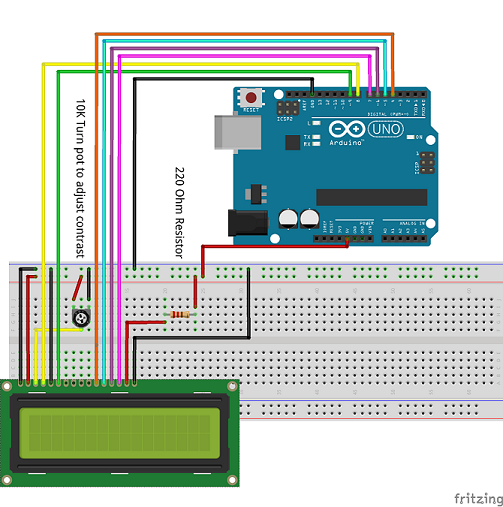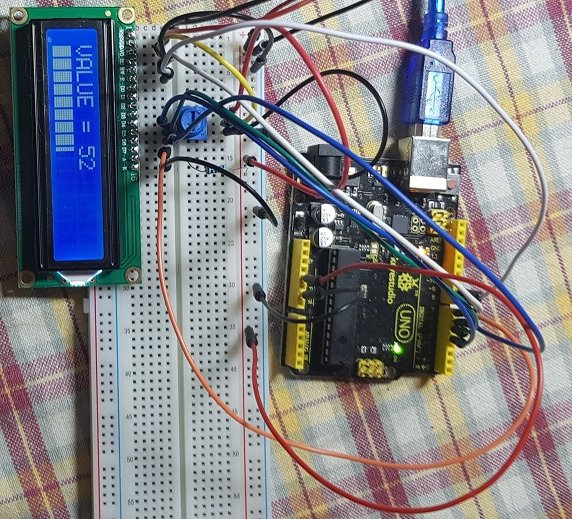# B4R TutorialLCD Bar Graph

The attached project mimics this posting. Have added the basic functionality with inline C. It uses the rCore and rLiquidCrystal libraries.

Wiring diagram:Result:Sample code (change it to your licking):
B4X:
``````'LCD Display - LCD 1602
'See attached wiring diagram

#Region Project Attributes
#AutoFlushLogs: True
#CheckArrayBounds: True
#StackBufferSize: 300
#End Region

Sub Process_Globals
'These global variables will be declared once when the application starts.
'Public variables can be accessed from all modules.
Public Serial1 As Serial
Dim lcd As LiquidCrystal
Dim t As Timer
Dim maxvalue As Byte = 100

End Sub

Private Sub AppStart
Serial1.Initialize(115200)
Log("AppStart")

t.Initialize("t_Tick", 500)
lcd.Initialize(8,255,9, Array As Byte(4,5,6,7))
Delay(10)
lcd.Begin(16, 2)
lcd.Clear

RunNative("createOne",Null)
RunNative("createTwo",Null)
RunNative("createThree",Null)
RunNative("createFour",Null)
RunNative("createFive",Null)

lcd.SetCursor(0,0)

lcd.Write("B4R - LCD Graph")
Delay(3000)
lcd.SetCursor(0,1)
Delay(10)
RunNative("writeCharAlarm", 0)

t.Enabled = True

End Sub

Sub t_Tick

Dim i As Byte = Rnd(0, maxvalue + 1)
lcd.Clear
lcd.SetCursor(0,0)
lcd.Write("VALUE = ")
lcd.SetCursor(8,0)
lcd.Write(i)

lcd.SetCursor(0,1)

Dim full As Float = 16 * (i/maxvalue)
Dim ff As Int = full

Dim fraction As Int = Round(10 * (full - ff))
fraction = Round((fraction * 5 / 10))

For j = 1 To full
RunNative("writeCharAlarm", 4)
Next

If fraction = 1 Then
RunNative("writeCharAlarm", 0)
Else if fraction = 2 Then
RunNative("writeCharAlarm", 1)
Else if fraction = 3 Then
RunNative("writeCharAlarm", 2)
Else if fraction = 4 Then
RunNative("writeCharAlarm", 3)

End If

End Sub

#if C

Byte One = {
B10000,
B10000,
B10000,
B10000,
B10000,
B10000,
B10000,
B10000
};

byte Two = {
B11000,
B11000,
B11000,
B11000,
B11000,
B11000,
B11000,
B11000
};

Byte Three = {
B11100,
B11100,
B11100,
B11100,
B11100,
B11100,
B11100,
B11100
};

Byte Four = {
B11110,
B11110,
B11110,
B11110,
B11110,
B11110,
B11110,
B11110
};

Byte Five = {
B11111,
B11111,
B11111,
B11111,
B11111,
B11111,
B11111,
B11111
};

void createOne(B4R::Object* o) {
b4r_main::_lcd->lc->createChar(0, One);
}

void createTwo(B4R::Object* o) {
b4r_main::_lcd->lc->createChar(1, Two);
}

void createThree(B4R::Object* o) {
b4r_main::_lcd->lc->createChar(2, Three);
}

void createFour(B4R::Object* o) {
b4r_main::_lcd->lc->createChar(3, Four);
}

void createFive(B4R::Object* o) {
b4r_main::_lcd->lc->createChar(4, Five);
}

void writeCharAlarm(B4R::Object* o) {
b4r_main::_lcd->lc->write((Byte)o->toULong());
}

#end if``````

There are most probably better ways to do this - but the lcd bar graph in the above/attached is working.

#### Attachments

• b4rLiquidCrystalDisplay.zip
1.5 KB · Views: 337

#### Johan Schoeman

##### Expert
Longtime User
Same wiring diagram as in post #1 above - it just takes away some of the unwanted flickering of the display while changing values. The below code will draw a bar graph in row 2 of the LCD display, starting at zero and going up to "maxvalue", and then down from maxvalue to zero (see comments added to the code) - actual value is displayed in Row 0 of the LCD display.

Change the value of "maxvalue" for a different "range"
B4X:
``````Sub Process_Globals
'These global variables will be declared once when the application starts.
'Public variables can be accessed from all modules.
....
....
....
Dim maxvalue As Int = 100                        'set the maximum value to display
....
....

End Sub``````

B4X:
``````#Region Project Attributes
#AutoFlushLogs: True
#CheckArrayBounds: True
#StackBufferSize: 300
#End Region

Sub Process_Globals
'These global variables will be declared once when the application starts.
'Public variables can be accessed from all modules.
Public Serial1 As Serial
Dim lcd As LiquidCrystal
Dim t As Timer                                   'create a timer
Dim maxvalue As Int = 100                        'set the maximum value to display
Dim i As Byte
Dim countupwards As Boolean = True               'are we counting up of down....

End Sub

Private Sub AppStart
Serial1.Initialize(115200)
Log("AppStart")

t.Initialize("t_Tick", 200)                             'initialize the timer
lcd.Initialize(8,255,9, Array As Byte(4,5,6,7))         'using pins 4, 5, 6, 7 for data, pin 8 = RS, pin 9 = Enable
Delay(10)
lcd.Begin(16, 2)                                        'set LCD display for 16 columns and 2 rows
lcd.Clear

RunNative("createOne",Null)                             'create the character that displays one column of 8 pixels (on LCD 1602 there are 16 * 5 = 80 columns)
RunNative("createTwo",Null)                             'create the character that displays two columns of 8 pixels
RunNative("createThree",Null)                           'create the character that displays three columns of 8 pixels
RunNative("createFour",Null)                            'create the character that displays four columns of 8 pixels
RunNative("createFive",Null)                            'create the character that displays five columns of 8 pixels

lcd.SetCursor(0,0)                                      'set the cursor to column 0, row 0

lcd.Write("B4R - LCD Graph")                            'display initial message
Delay(1500)                                             'show initial message for 1.5 seconds
lcd.Clear                                               'clear the display
lcd.SetCursor(0,1)                                      'set the cursor to column 0, row 1

t.Enabled = True                                        'enable the time

End Sub

Sub t_Tick

lcd.SetCursor(0,0)                                      'set cursor to column 0, row 0
lcd.Write("VALUE = ")                                   'display "VALUE = "
For k = 8 To 15                                         'write a space (" ") to the remainder colums of row 0
lcd.SetCursor(k,0)
lcd.Write(" ")                                      'write the space
Next
lcd.SetCursor(8,0)                                      'set the cursor to column 8, row 0 (rows and coluns start at 0)
lcd.Write(i)                                            'write the value of i to the LCD - it will display for eg  "VALUE = 73"

lcd.SetCursor(0,1)                                      'put the cursor at column 0, row 1 (preparing for the bar graph)

Log("i = ", i)
Dim full As Float = 16 * (i/maxvalue)                   'calculate how many chars of 5x8 are needed to draw the bar graph (eg if maxvalue = 100, then if value to display = 73, then 16 * 73/100 is the number of "chars"on the LCD display to switch on")
Dim ff As Int = full                                    'get the INT number of full 5x8 chars to switch on
Log("ff = ", ff)

Dim fraction As Int = Round(10 * (full - ff))           'calculate the fraction of a "char" that must be switched on
fraction = Round((fraction * 5 / 10))                   'eg if calue to display = 73 and maxvalue = 100, 73/100 = 0.73 * 16 = 11 full chars and 0.68 of a char
Log("fraction = ", fraction)                            '0.68 of a char  = 0.68 * 5 = 3.4 (then rounding 3.4 to the nearest int)
Log(" ")

lcd.SetCursor(0,1)                                      'first, fill the number of full chars with 5*8 all switched on
For j = 0 To ff - 1
RunNative("writeCharAlarm", 4)                      'write the nuber of full chars
Next

If fraction = 1 Then                                    'now write the fractions
RunNative("writeCharAlarm", 0)                      'it can be 1 up to 4 rows of 8 (column) pixels - on a 16 * x display there 80 pixel columns
Else if fraction = 2 Then
RunNative("writeCharAlarm", 1)
Else if fraction = 3 Then
RunNative("writeCharAlarm", 2)
Else if fraction = 4 Then
RunNative("writeCharAlarm", 3)
Else if fraction = 5 Then
RunNative("writeCharAlarm", 4)
Else if fraction = 0 Then
End If

Dim dummy As Int = 0                                   'want to determine where to clear the bar graph from without having to call lcd.Clear - it makes the display less flickering
If fraction > 0 Then
dummy = 1
Else
dummy = 0
End If

Dim total_on As Int = ff + dummy                       'how many chars to clear in the bargraph after new bar graph has been written to the display

For k = total_on To 15                                 'clear the excess "chars" in the bar graph
If total_on < 16 Then
lcd.SetCursor(k, 1)
lcd.Write(" ")
End If
Next

If countupwards = True Then
count_up                                           'count up from 0 to maxvalue and switch direction when getting to maxvalue - bar graph will follow suite
Else
count_down                                           'count down from maxvalue to 0 and switch direction when getting to maxvalue - bar graph will follow suite
End If

End Sub

Sub count_up                                             'when counting up

i = i + 1
If i = maxvalue Then
Delay(100)
countupwards = Not(countupwards)                 'switch counting direction
End If

End Sub

Sub count_down                                            'when counting down

i = i - 1
If i = 0 Then
Delay(100)
countupwards = Not(countupwards)                  'switch counting direction
End If

End Sub

'the C code below creates the custom chars and displays them
#if C

Byte One = {
B10000,
B10000,
B10000,
B10000,
B10000,
B10000,
B10000,
B10000
};

byte Two = {
B11000,
B11000,
B11000,
B11000,
B11000,
B11000,
B11000,
B11000
};

Byte Three = {
B11100,
B11100,
B11100,
B11100,
B11100,
B11100,
B11100,
B11100
};

Byte Four = {
B11110,
B11110,
B11110,
B11110,
B11110,
B11110,
B11110,
B11110
};

Byte Five = {
B11111,
B11111,
B11111,
B11111,
B11111,
B11111,
B11111,
B11111
};

//create ONE = one column ON (out of 80 columns on a 16*2 LCD display with 5 rows x 8 pixels per LCD char)
void createOne(B4R::Object* o) {
b4r_main::_lcd->lc->createChar(0, One);
}

//create TWO = two columns ON (out of 80 columns on a 16*2 LCD display with 5 rows x 8 pixels per LCD char)
void createTwo(B4R::Object* o) {
b4r_main::_lcd->lc->createChar(1, Two);
}

//create THREE = three columns ON (out of 80 columns on a 16*2 LCD display with 5 rows x 8 pixels per LCD char)
void createThree(B4R::Object* o) {
b4r_main::_lcd->lc->createChar(2, Three);
}

//create FOUR = four columns ON (out of 80 columns on a 16*2 LCD display with 5 rows x 8 pixels per LCD char)
void createFour(B4R::Object* o) {
b4r_main::_lcd->lc->createChar(3, Four);
}

//create FIVE = five columns ON (out of 80 columns on a 16*2 LCD display with 5 rows x 8 pixels per LCD char)
void createFive(B4R::Object* o) {
b4r_main::_lcd->lc->createChar(4, Five);
}

//dispaly the char (0 to 4) that has been created and stored in the LCD Display
void writeCharAlarm(B4R::Object* o) {
b4r_main::_lcd->lc->write((Byte)o->toULong());
}

#end if``````

•Mostez